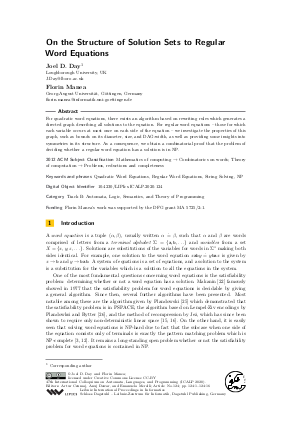Document# On the Structure of Solution Sets to Regular Word Equations

### Authors Joel D. Day, Florin Manea## File

LIPIcs.ICALP.2020.124.pdf
• Filesize: 0.57 MB
• 16 pages

## Cite As

Joel D. Day and Florin Manea. On the Structure of Solution Sets to Regular Word Equations. In 47th International Colloquium on Automata, Languages, and Programming (ICALP 2020). Leibniz International Proceedings in Informatics (LIPIcs), Volume 168, pp. 124:1-124:16, Schloss Dagstuhl - Leibniz-Zentrum für Informatik (2020)
https://doi.org/10.4230/LIPIcs.ICALP.2020.124

## Abstract

For quadratic word equations, there exists an algorithm based on rewriting rules which generates a directed graph describing all solutions to the equation. For regular word equations - those for which each variable occurs at most once on each side of the equation - we investigate the properties of this graph, such as bounds on its diameter, size, and DAG-width, as well as providing some insights into symmetries in its structure. As a consequence, we obtain a combinatorial proof that the problem of deciding whether a regular word equation has a solution is in NP.

## Subject Classification

##### ACM Subject Classification
• Mathematics of computing → Combinatorics on words
• Theory of computation → Problems, reductions and completeness
##### Keywords
• Regular Word Equations
• String Solving
• NP

## Metrics

• Access Statistics
• Total Accesses (updated on a weekly basis)
0

## References

1. P. A. Abdulla, M. F. Atig, Y. Chen, L. Holík, A. Rezine, P. Rümmer, and J. Stenman. Norn: An SMT solver for string constraints. In Proc. Computer Aided Verification (CAV), volume 9206 of Lecture Notes in Computer Science (LNCS), pages 462-469, 2015.2. M. Alkhalaf, T. Bultan, and F. Yu. STRANGER: An automata-based string analysis tool for PHP. In Proc. Tools and Algorithms for the Construction and Analysis of Systems (TACAS), volume 6015 of Lecture Notes in Computer Science (LNCS), 2010.3. D. Angluin. Finding patterns common to a set of strings. Journal of Computer and System Sciences, 21:46-62, 1980.4. C. Barrett, C. L. Conway, M. Deters, L. Hadarean, D. Jovanović, T. King, A. Reynolds, and C. Tinelli. CVC4. In Proc. Computer Aided Verification (CAV), volume 6806 of Lecture Notes in Computer Science (LNCS), pages 171-177, 2011.5. D. Berwanger, A. Dawar, P. Hunter, S. Kreutzer, and J. Obdrzálek. The DAG-width of directed graphs. Journal of Combinatorial Theory, Series B, 102(4):900-923, 2012.6. M. Berzish, V. Ganesh, and Y. Zheng. Z3str3: A string solver with theory-aware heuristics. In Proc. Formal Methods in Computer-Aided Design (FMCAD), pages 55-59. IEEE, 2017.7. J. D. Day, V. Ganesh, P.l He, F. Manea, and D. Nowotka. The satisfiability of word equations: Decidable and undecidable theories. In I. Potapov and P. Reynier, editors, In Proc. 12th International Conference on Reachability Problems, RP 2018, volume 11123 of Lecture Notes in Computer Science (LNCS), pages 15-29, 2018.8. J. D. Day, F. Manea, and D. Nowotka. The hardness of solving simple word equations. In Proc. Mathematical Foundations of Computer Science (MFCS), volume 83 of LIPIcs, pages 18:1-18:14, 2017.9. J. D. Day, F. Manea, and D. Nowotka. Upper bounds on the length of minimal solutions to certain quadratic word equations. In Proc. Mathematical Foundations of Computer Science (MFCS), volume 138 of LIPIcs, pages 44:1-44:15, 2019.10. V. Diekert, A. Jeż, and W. Plandowski. Finding all solutions of equations in free groups and monoids with involution. Information and Computation, 251:263-286, 2016.11. V. Diekert and J. M. Robson. On quadratic word equations. In Proc. 16th Annual Symposium on Theoretical Aspects of Computer Science, STACS, volume 1563 of Lecture Notes in Computer Science (LNCS), pages 217-226, 1999.12. A. Ehrenfeucht and G. Rozenberg. Finding a homomorphism between two words is NP-complete. Information Processing Letters, 9:86-88, 1979.13. D. D. Freydenberger. A logic for document spanners. Theory of Computing Systems, 63(7):1679-1754, 2019.14. D. D. Freydenberger and M. Holldack. Document spanners: From expressive power to decision problems. Theory of Computing Systems, 62(4):854-898, 2018.15. A. Jeż. Recompression: A simple and powerful technique for word equations. Journal of the ACM, 63, 2016.16. A. Jeż. Word equations in nondeterministic linear space. In Proc. International Colloquium on Automata, Languages and Programming (ICALP), volume 80 of LIPIcs, pages 95:1-95:13, 2017.17. J. Karhumäki, F. Mignosi, and W. Plandowski. The expressibility of languages and relations by word equations. Journal of the ACM, 47:483-505, 2000.18. A. Kiezun, V. Ganesh, P. J. Guo, P. Hooimeijer, and M. D. Ernst. HAMPI: a solver for string constraints. In Proc. ACM SIGSOFT International Symposium on Software Testing and Analysis (ISSTA), pages 105-116. ACM, 2009.19. A. W. Lin and P. Barceló. String solving with word equations and transducers: towards a logic for analysing mutation xss. In ACM SIGPLAN Notices, volume 51, pages 123-136. ACM, 2016.20. A. W. Lin and R. Majumdar. Quadratic word equations with length constraints, counter systems, and Presburger arithmetic with divisibility. In S. K. Lahiri and C. Wang, editors, In Proc. 16th International Symposium on Automated Technology for Verification and Analysis (ATVA), volume 11138 of Lecture Notes in Computer Science (LNCS), pages 352-369. Springer, 2018.21. M. Lothaire. Algebraic Combinatorics on Words. Cambridge University Press, Cambridge, New York, 2002.22. G. S. Makanin. The problem of solvability of equations in a free semigroup. Sbornik: Mathematics, 32(2):129-198, 1977.23. F. Manea, D. Nowotka, and M. L. Schmid. On the complexity of solving restricted word equations. International Journal of Foundations of Computer Science, 29(5):893-909, 2018.24. E. Petre. An elementary proof for the non-parametrizability of the equation xyz = zvx. In Proc. 29th International Symposium on Mathematical Foundations of Computer Science (MFCS), volume 3153 of Lecture Notes in Computer Science (LNCS), pages 807-817, 2004.25. W. Plandowski. Satisfiability of word equations with constants is in PSPACE. In Proc. Foundations of Computer Science (FOCS), pages 495-500. IEEE, 1999.26. W. Plandowski and W. Rytter. Application of Lempel-Ziv encodings to the solution of words equations. In Proc. International Colloquium on Automata, Languages and Programming (ICALP), volume 1443 of Lecture Notes in Computer Science (LNCS), pages 731-742, 1998.27. K. U. Schulz. Makanin’s algorithm for word equations-two improvements and a generalization. In International Workshop on Word Equations and Related Topics, pages 85-150. Springer, 1990.X

Feedback for Dagstuhl Publishing